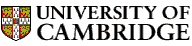Computer LaboratoryInformation Theory and Coding# Information Theory and Coding2004-05

Principal lecturer: Dr John Daugman
Taken by: Part II

Prerequisite courses: Continuous Mathematics, Probability, Discrete Mathematics

Number of lectures: 16 (Tues, Thurs at 12, Lecture Theatre 2)

#### Aims

The aims of this course are to introduce the principles and applications of information theory. The course will study how information is measured in terms of probability and entropy, and the relationships among conditional and joint entropies; how these are used to calculate the capacity of a communication channel, with and without noise; coding schemes, including error correcting codes; how discrete channels and measures of information generalize to their continuous forms; the Fourier perspective; and extensions to wavelets, complexity, time series, compression, and efficient coding of audio-visual information for human perception.

#### Lectures

• Overview and historical origins: foundations and uncertainty. Why the movements and transformations of information, just like those of a fluid, are law-governed. How concepts of randomness, redundancy, compressibility, noise, bandwidth, and uncertainty are intricately connected to information. Origins of these ideas and the various forms that they take.

• Mathematical foundations; probability rules; Bayes' theorem. The meanings of probability. Ensembles, random variables, marginal and conditional probabilities. How the formal concepts of information are grounded in the principles and rules of probability.

• Entropies defined, and why they are measures of information. Marginal entropy, joint entropy, conditional entropy, and the Chain Rule for entropy. Mutual information between ensembles of random variables. Why entropy is the fundamental measure of information content.

• Source coding theorem; prefix, variable-, and fixed-length codes. Symbol codes. The binary symmetric channel. Capacity of a noiseless discrete channel. Error correcting codes.

• Channel types, properties, noise, and channel capacity. Perfect communication through a noisy channel. Capacity of a discrete channel as the maximum of its mutual information over all possible input distributions.

• Continuous information; density; noisy channel coding theorem. Extensions of the discrete entropies and measures to the continuous case. Signal-to-noise ratio; power spectral density. Gaussian channels. Relative significance of bandwidth and noise limitations. The Shannon rate limit and efficiency for noisy continuous channels.

• Fourier series, convergence, orthogonal representation. Generalised signal expansions in vector spaces. Independence. Representation of continuous or discrete data by complex exponentials. The Fourier basis. Fourier series for periodic functions. Examples.

• Useful Fourier theorems; transform pairs. Sampling; aliasing. The Fourier transform for non-periodic functions. Properties of the transform, and examples. Nyquist's Sampling Theorem derived, and the cause (and removal) of aliasing.

• Discrete Fourier transform. Fast Fourier Transform Algorithms. Efficient algorithms for computing Fourier transforms of discrete data. Computational complexity. Filters, correlation, modulation, demodulation, coherence.

• The quantised degrees-of-freedom in a continuous signal. Why a continuous signal of finite bandwidth and duration has a fixed number of degrees-of-freedom. Diverse illustrations of the principle that information, even in such a signal, comes in quantised, countable, packets.

• Gabor-Heisenberg-Weyl uncertainty relation. Optimal ``Logons''. Unification of the time-domain and the frequency-domain as endpoints of a continuous deformation. The Uncertainty Principle and its optimal solution by Gabor's expansion basis of ``logons''. Multi-resolution wavelet codes. Extension to images, for analysis and compression.

• Kolmogorov complexity and minimal description length. Definition of the algorithmic complexity of a data sequence, and its relation to the entropy of the distribution from which the data was drawn. Shortest possible description length, and fractals.

• Correlation coding. Random vectors, dependence versus correlation, covariance, decorrelation, matrix diagonalisation, eigen decomposition, Karhunen-Loeve transform, principal/independent components analysis. Relation to orthogonal transform coding using fixed basis vectors, such as Discrete Cosine Transform.

• Lossy versus lossless compression. What information is discarded by human senses and can be eliminated by encoders. Perceptual scales, masking, spatial resolution, colour coordinates; some demonstration experiments.

• Quantization; image and audio coding standards. JPEG photographic still-image compression, motion compensation, MPEG video encoding, MPEG audio encoding. A/u-law coding, delta coding, linear predictive coding, voice compression, vector quantisation. (2 lectures)

#### Objectives

At the end of the course students should be able to

• calculate the information content of a random variable from its probability distribution

• relate the joint, conditional, and marginal entropies of variables in terms of their coupled probabilities

• define channel capacities and properties using Shannon's Theorems

• construct efficient codes for data on imperfect communication channels

• generalise the discrete concepts to continuous signals on continuous channels

• understand Fourier Transforms and the main ideas behind efficient algorithms for them

• describe the information resolution and compression properties of wavelets

• understand and explain the limits in human perception that are exploited for irrelevance-reduction coding

• provide a good overview of the principles and characteristics of several widely-used compression techniques and standards for audio-visual signals, and know how to select an appropriate compression algorithm

#### Recommended book

Cover, T.M. & Thomas, J.A. (1991). Elements of Information Theory. New York: Wiley.

Classical paper by Claude Shannon (1948) for reference: "A Mathematical Theory of Communication"© 2004 Dr John Daugman & Dr Neil Dodgson Please send any comments to pagemaster@cl.cam.ac.uk